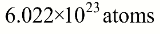How many molecules are there in 220 grams of ?

# How many molecules are there in 220 grams of?

1. A2. B3. C4. D
None of the above

Fill Out the Form for Expert Academic Guidance!l

+91

Live ClassesBooksTest SeriesSelf Learning

Verify OTP Code (required)

### Solution:

Concept-Molar mass ofis.Number of particles in$×$molecules.
Hence, the correct answer is option 1).

## Related content

 Area of Square Area of Isosceles Triangle Pythagoras Theorem Triangle Formula Perimeter of Triangle Formula Area Formulae Volume of Cone Formula Matrices and Determinants_mathematics Critical Points Solved Examples Type of relations_mathematics+91

Live ClassesBooksTest SeriesSelf Learning

Verify OTP Code (required)